Contact Us

E i n s t e i n E l e c t r i c i t y . c o m

Einstein's   Biggest   Pursuit   Finally   Discovered !

The Connection Between Gravity And Electricity.

Most  Important;

Before we're even able to comprehend the origin of Electricity we need to fully understand the TRUE origin of Gravity.  Gravity is absolutely the flow of quantum particles of mass "into" the earth.  q-Particles, which are the building blocks of Space (yes space is NOT empty) are flowing from space into the earth (becoming earth Mass).  And this is exactly what is right now Pushing you down onto the earth = Gravity.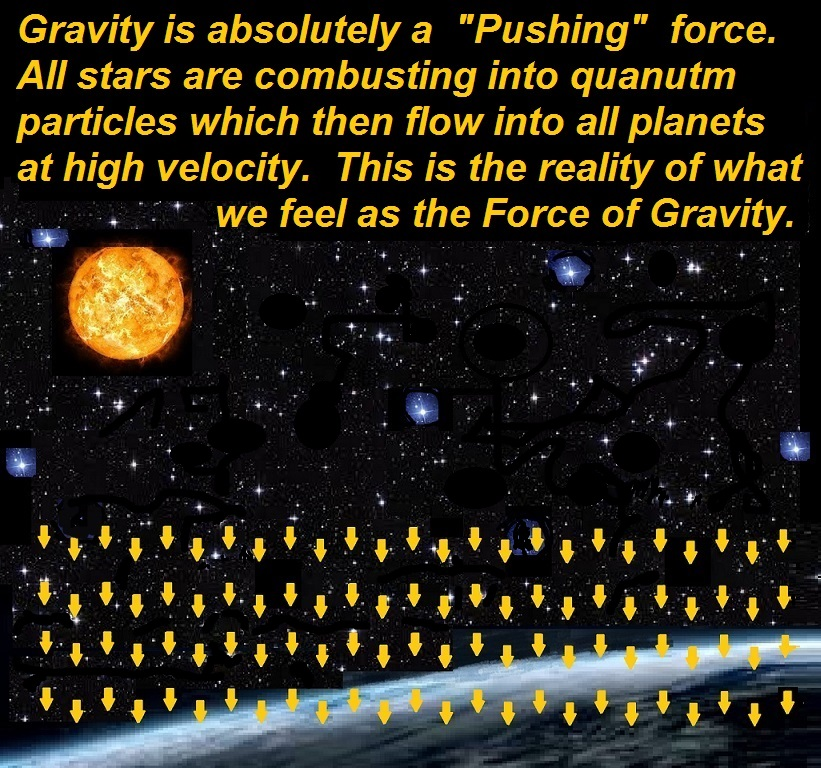We can understand the relationship between Gravity and Electricity best

when we realize the exact parallels between these  3  scenarios below;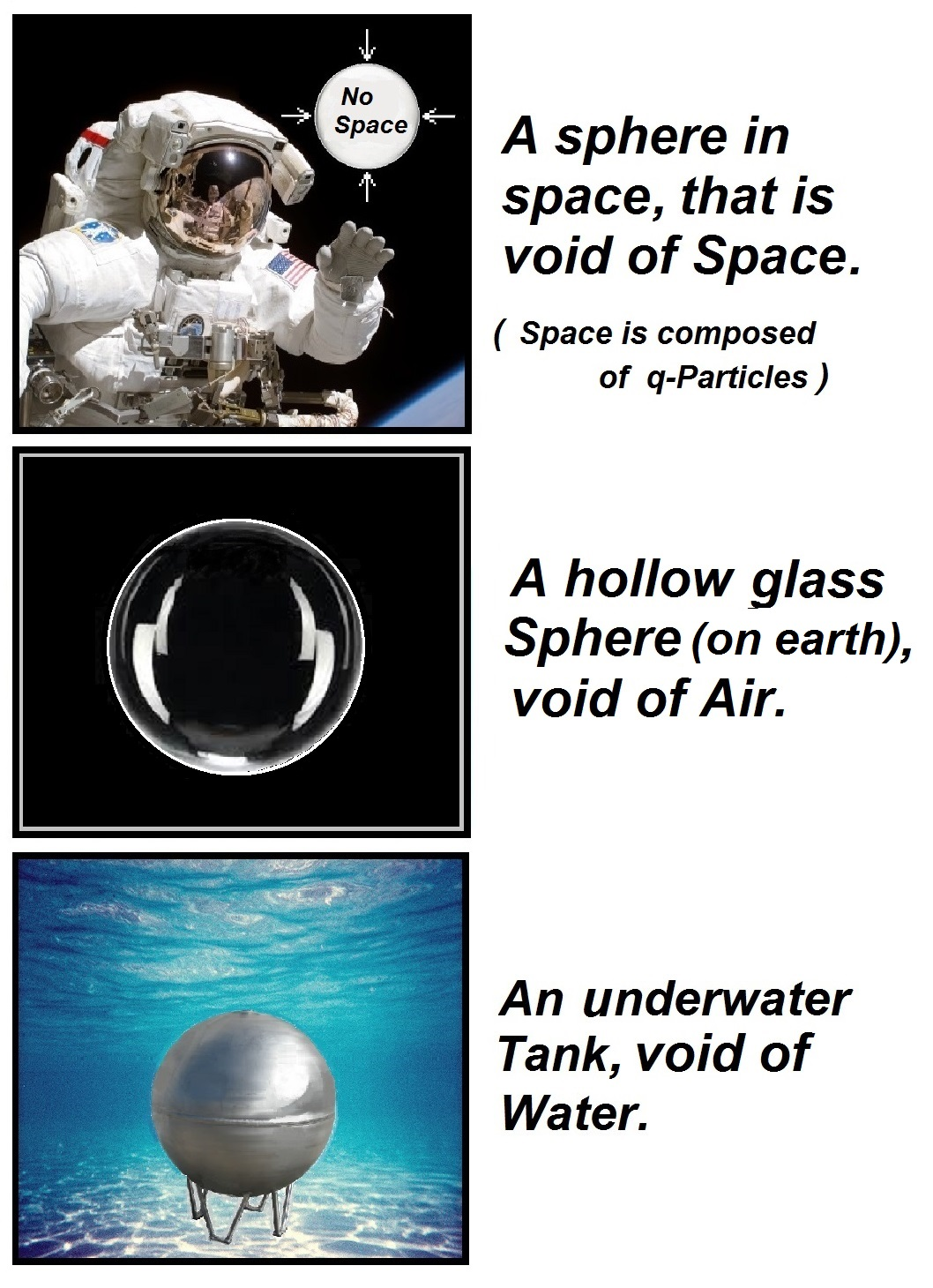Gravity is absolutely a  "Pushing"  force !

And it pushes Electricity at its own same Velocity !

In the image below, Gravity is flowing through the Magnets (must see  www.EinsteinMagnetism.com) and as the rotor turns the free electrons on the coil are being pushed.  And if the current cannot flow, then Gravity simply backs up and creates a low pressure "Energy Potential Sphere" (the true definition of a capacitor).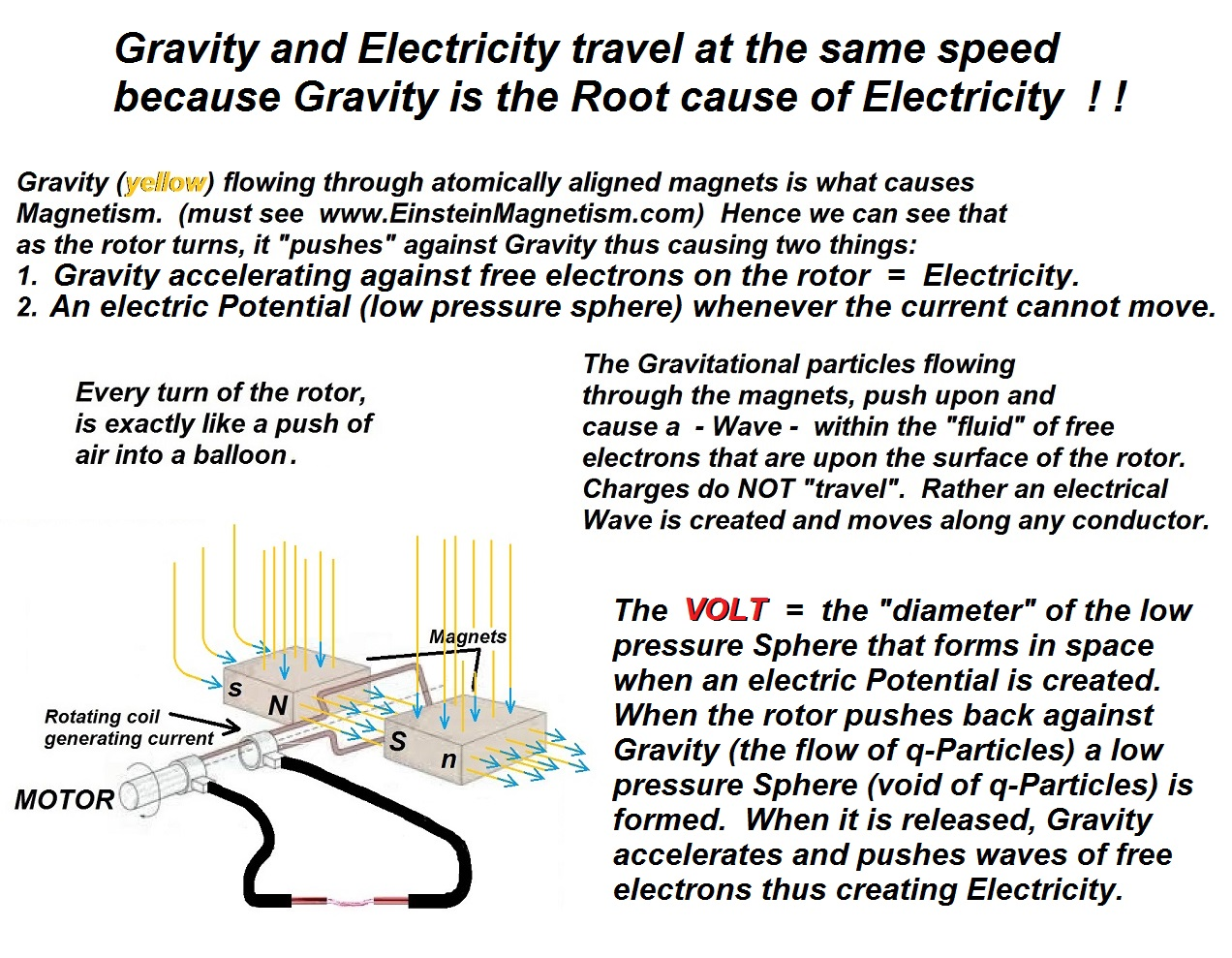When an electric generator creates an electric Volt Potential, it is simply creating within Space, a spherical void of  "Flowing Gravity".

We need to understand that "Space" is NOT empty !

The diameter of the above low pressure "Gravity sphere in space" is the exact  physical  definition of the  VOLT.  The above sphere within space (outerSpace as well as Space within our atmosphere) is a true Capacitor.  With a physical capacitor, the flowing Gravity becomes built up on the physical plates exactly like a water dam on earth.  And when an electrical connection is complete, the higher pressure Gravity then flows and creates waves of free electrons = Electricity.  In two simple terms we need to understand that;

Pushing back against the flow of Gravity  =  an Electric Volt Potential.

and

Gravity accelerating against free electrons  =  Electricity.

(The constant factor of flowing Gravity is unnoticeable,

only "Accelerated Flow" is noticeable  =  Electricity.)

But one of the biggest things we need to realize, is that;

Today's  Fundamental  Electrical  Model  Has

An  Enormous  Error.

Electricity is not the "movement of Charges".  Coulombs do not  "travel" along a conductor and thus create electricity.

Rather, free electrons that are in a "fluid state" upon the surface of any conductor, are Accelerated and that acceleration creates an electrical wave that travels along any wire.

This wave travels along the surface of the wire between the wire and the opposing Pressure of space which surrounds all conductors.  The degree to which any metal is a good conductor is determined by the "depth and viscosity" of that free electron fluid which is on the surface of any conductor.

VIEW  THIS  IMAGE

In the above animated gif we see the free electron from a side view orbiting.  The electron is being Accelerated and thus producing a  WAVE  that travels along the wire.

When this reality is embraced, the two erroneous electrical SI entities, namely;

Coulomb (Charge)

Volt (Joule/Coulomb)  are then translated into their true SI units of;

Coulomb  =  mass x Acceleration  (mA)  force of Space Pressure.

Volt           =  Distance (AZ2) of the Diameter of the Pressure Sphere.

The above two units are exactly proportional to;

Coulomb  =  The Accelerated collapse of the "true void" sphere in space Pressure which is the reality of an "Electrical Potential".

Volt           =  The Diameter distance of the spherical "true void" in space that is created by either running a magnet through a coil, or burning mass.

Below is the beginning of a  TRUE  understanding of Electricity.

In the chart below, the third column must be read using

the 3 fundamentals of Electricity;

m = Mass          A = Acceleration          Z = Time

 The reason that Electrical Physics has been hindered so badly has been from the fact that the "Amp" below is not described in a True physical reality. m=meter A=Ampere s=Time The True physical Electrical equations below are; m = Mass A = Acceleration Z = Time Joule kg m2 s−2 mA2Z2 Watt kg m2 s−3 mA2Z Coulomb A s mA Volt kg m2 s−3 A−1 AZ2 Amp Coulomb / Second mA / Z Farad kg−1 m−2 s4 A2 m / Z2 Ohm kg m2 s−3 A−2 Z3 / m Siemens kg−1 m−2 s3 A2 m / Z3 Weber kg m2 s−2 A−1 AZ3 Tesla kg s−2 A−1 1 / AZ Henry kg m2 s−2 A−2 Z4 / m El. Field Volt / Meter AZ2 / meter

Further scientific proof is unveiled via earth orbit Energy  =  mv2.  You can see in the above chart that the Tesla is defined as  1/ Velocity (1/AZ).  Wikipedia states that the Magnetic Field (Tesla) of the earth  =  .000032  When you divide  1  by earth orbit Velocity (1/AZ) you get  .000032  Teslas.

The  Connection  Between  Gravity  And  Electricity  Finally  Discovered.

For the last  30 years of his life Albert Einstein diligently struggled to connect Gravity and Electricity.  Below is the indisputable Mathematical Proof that Einstein searched for the last 20 years of his life.

The Velocity factor "v" in the energy equation  E=mv2  must be reduced to its core factors of (Acceleration x Time) which are the building blocks of Velocity.  And because Einstein did not do this in, all of the historically known Electrical entities (Volt, Amp, Ohm, etc) that are housed "within" the one true equation for Energy were then kept hidden from Einstein.

E = mv2

Energy = mass x (Velocity)2

more precisely

Energy = mass x (Acceleration x Time)2

m = Mass          A = Acceleration          Z = Time

hence the one true equation for Electrical Energy becomes;

E = mv2

E = m(AZ)2

E = mA2Z2

Very  Important ;

The Velocity of Light = The Acceleration of Gravity x earth's Orbit Time.  Earth's Gravity (9.8 m/s2)  x  earth's Orbit Time (30591067.1428571429 seconds, exact lunar year)  =  the Velocity of Light  299,792,458 m/s.  Gravity is the Pressure of quantum-Mass originating from the sun that is right now pushing us down onto the earth.  If a person implements the traditional (erroneous) "pulling" definition of Gravity (or Einstein's curved space-time) into the following algebra they will only induce needless confusion to themselves and others.  Please do not implement today's mistaken (pulling) Gravity into the following mathematically proven truth.

All of the Electrical equations below have been proven a long time ago via

Electrical Engineering and hundreds of years of scientific history.

 mA2Z2 m=Mass  A=Accel.  Z=Time kg m2 / s2 kg=Mass  m=Meter  s=Time

 Joule  = mA2Z2 kg m2 / s2 Coulomb  x  Volt Amp  x  Weber Watt  x  Time

 Watt  = mA2Z kg m2 / s3 Amp x Volt Amp2  x  Ohm Volt2  /  Ohm

 Ampere  = mA/Z kg meter / s3 Watt  /  Volt Volt  /  Ohm √Watt  /  Ohm

 Volt  = AZ2 meter Amp  x  Ohm Joule  /  Coulomb Watt  /  Amp √Watt  x  Ohm

 Ohm  = Z3/m s3 / kg Volt  /  Amp Watt  /  Amp2 Inverse  Siemens

 Coulomb  = mA kg meter / s2 Amp  x  Time Joule  /  Volt Volt  x  Farad

 Farad  = m/Z2 kg / s2 Joule  /  Volt2 Coulomb  /  Volt Coulomb2  /  Joule

 Weber  = AZ3 meter second Volt  x  Time Henry  x  Amp Coulomb x Ohm

 Tesla  = 1/AZ second / meter Weber  /  Meter2 Volt  x  Sec  /  Meter2 Volt  /  Amp2  x  Sec

 Siemens  = m/Z3 kg / s3 Amp  /  Volt Farad  /  Time Inverse Ohm

 Elec. Field  = Volt/Meter distance / meter Newton  /  Coulomb Ohm  x  Siemens Volt x Time / Weber

 Henry  = Z4/m s4 / kg Ohm x Time Volt x Time / Amp Joule x Time2  / Coulomb2

The fact that all of the blue derivatives below;

 Energy  =  m(AZ)2 Joule  =  mA2Z2 Watt  =  mA2Z Coulomb  =  mA Volt  =  AZ2 Amp  =  mA/Z Weber  =  AZ3 Farad  =  m/Z2 Ohm  =  Z3/m Tesla  =  1/AZ Siemens  =  m/Z3 El. Field  =  Volt/Meter = 1/1 Henry  =  Z4/m Acceleration  =  A Time  =  Z

perfectly harmonize with each and every electrical entity below is no coincidence.  It is absolute proof to the unification of Gravity, Electricity, and Einstein's  E=mc2 Please understand, if this information was not true, the equational synchronization below would simply be a mathematical impossibility.  Be sure to pay close attention to the fact that 4 independent demands are being fulfilled within the equations below;

Multiplication,     Division,     Square Root,     X2

All four entities engaging in perfect sync.

 Joule  = Energy Coulomb  x  Volt Amp  x  Weber Watt  x  Time m(AZ)2  = mA2Z2 mA  x  AZ2 qA/Z  x  AZ3 mA2Z  x  Z

 Watt  = Amp  x  Volt Amp2  x  Ohm Volt2  /  Ohm mA2Z  = mA/Z  x  AZ2 m2A2/Z2  x  Z3/m A2Z4  /  Z3/m

 Ampere  = Watt  /  Volt Volt  /  Ohm √Watt  /  Ohm mA/Z  = mA2Z  /  AZ2 AZ2  /  Z3/m √mA2Z  /  Z3/m

 Volt  = Amp  x  Ohm Joule  /  Coulomb Watt  /  Amp √Watt  x  Ohm AZ2  = mA/Z  x  Z3/m mA2Z2  /  mA mA2Z  /  mA/Z √mA2Z  x  Z3/m

 Ohm  = Volt  /  Amp Watt  /  Amp2 Inverse  Siemens Z3/m  = AZ2  /  mA/Z mA2Z  /  m2A2/Z2 1  /  Z3  /  m

 Coulomb  = Amp  x  Time Joule  /  Volt Volt  x  Farad mA  = mA/Z  x  Z mA2Z2  /  AZ2 AZ2  x  m/Z2

 Farad  = Joule  /  Volt2 Coulomb  /  Volt Coulomb2  /  Joule m/Z2  = mA2Z2  /  A2Z4 mA  /  AZ2 m2A2  /  mA2Z2

 Weber  = Volt  x  Time Henry  x  Amp Coulomb  x  Ohm AZ3  = AZ2  x  Z Z4/m  x  mA/Z mA  x  Z3/m

 Tesla  = Weber  /  Meter2 Volt  x  Sec  /  Meter2 Volt  /  Amp2  x  Sec 1/AZ  = AZ3  /  A2Z4 AZ2  x  Z  /  A2Z4 AZ2  /  m2A2/Z2  x  Z

 Siemens  = Amp  /  Volt Farad  /  Time Inverse Ohm m/Z3  = mA/Z  /  AZ2 m/Z2  /  Z 1  /  Z3/m

 Electric Field  = Newton  /  Coulomb Ohm  x  Siemens Volt  x  Time / Weber Volt/Meter  =  1/1 mA  /  mA Z3/m  x  m/Z3 AZ2 x Z  /  AZ3

 Henry  = Ohm x Time Volt x Time / Amp Joule x Time2 / Coulomb2 Z4/m  = Z3/m  x  Z AZ2  x  Z  /  mA/Z mA2Z2  x  Z2  /  m2A2

 Acceleration  = Volt  /  Time2 Inv. Volt  /  Tesla2 Watt /Coulomb x Time A  = AZ2  /  Z2 1 / AZ2 / 1 / A2Z2 mA2Z  /  mA  x  Z

 Time  = Volt  x  Tesla Coulomb  /  Amp Ohm  x  Farad Z  = AZ2  x  1/AZ mA  /  mA/Z Z3/m  x  m/Z2

For all of the above equations to unify completely as you can plainly see they do

would be simply  impossible  if

E = mA2Z2

were not the one and only true equation for Energy.

The above equation is the only equation that unifies Electricity, Gravity, Light, Magnetism, Quantum Mechanics, and Planetary Motion.

You should now return to

This page needs editing below

This page needs editing below

From Einstein's E=mc2 we get;

Energy = Mass x (Velocity of Light)2

Velocity = (Acceleration x Time)

Energy  =  Mass  x  (Acceleration  x  Time)2

A  =  Acceleration          Z  =  Time

Energy  =  m x A2 x Z2

Energy  =  mAZ  x  (Acceleration)  x  Time

And because the famous experiment of the physicist Willem Gravesande absolutely concluded that Energy was displayed via a piece of mass falling to the earth or (Mass x Gravitational Acceleration x Time), the red mAZ above must certainly represent Gravesande's Mass ball.  Therefore the "m" in mAZ must be redefined as "q" or Mass/Velocity = Electrical-Mass.

Therefore beyond question Einstein's "m" must = a building block of Mass.  Einstein's "m" = m/AZ.

m/AZ = q.

Einstein's "m" is certainly "Electrical-Mass" (q).

q x AZ = "Mass as we see it".

E = (qAZ) x AZ

E = q x AZ x AZ

the true Energy equation (unified with Gravity) is indeed;

E = q(AZ)2

E = qc2

Now when we look only at the  "numerical"  results that these two equations E=mc2 and E=qc2 both give us we will see that they are absolutely identical.  However when we dig deeper and look closely at the very physics difference of the two equations we will certainly discover a monumental and completely different story.  The story that declares exactly why one equation will unify with Gravity and the other could never do so in a million years.  And hence the very reason why E=mc2 caused Albert Einstein untold frustration for the last 30 years of his life.

Some sceptics do not understand the concept of Combinations and Permutations.

And the very real and certain law of;   Truth by Fact of Elimination.

The explanation below has only been expanded due to a couple of skeptics (claiming to be university degree educated) who contacted us and demanded that the rearranging of unit definitions does not provide proof whatsoever.  Well the reality is that they simply do not understand that an equation that fulfills "every" combination for every electrical value could not possibly do so unless it were the one and only true equation.

If  A  and  B  are two variables to an equation and  A x B  = 18  (only positive integers used) then there are only three sets of numbers that could possibly fulfill that equation;

1  and  18

( A x B = 18 )   ( 1 x 18 = 18 )

or

6  and  3

( A x B = 18 )   ( 6 x 3 = 18 )

or

9  and  2

( A x B = 18 )   ( 9 x 2 = 18 )

However if a second stipulation is now added, that

A2  divided by  B  must  =  12

then we can readily see that only the second set of numbers could fulfill the specific requirement, for only the numbers in the second set solution will produce the correct answer of

62 divided by 3  =  12.

This is exactly how electrical unification is proven.  Only an energy equation that completely coincides with all of the already historically proven electrical equations could possibly be true.  According to all of the proven equations below;

 E = qA2Z2   E = Energy    q = Quantum-Mass    A= Acceleration    Z = Time     Joule = (Watt x Time), (Volt x Coulomb), (qA2Z2)   Watt = (Volt x Amp), (Amp2 x Ohm), (Volt2 / Ohm), (qA2Z)   Ampere = (Watt / Volt), (Volt / Ohm), (√Watt / Ohm), (mA/Z)   Volt = (Amp x Ohm), (Joule / Coulomb), (Watt / Amp), (√Watt x Ohm), (AZ2)   Ohm = (Volt / Amp), (Watt / Amp2), (Joule / Amp2 x Time), (Z3/q)   Coulomb = (Amp x Time), (Joule / Volt), (Volt x Farad), (qA)   Farad = (Joule / Volt2), (Coulomb / Volt), (Coulomb2 / Joule), (q/Z2)   Weber = (Volt x Time), (Henry x Amp), (AZ3)   Tesla = (Weber / meter2), (Volt x Second / meter2), (1/AZ)  Siemens = (Time / Mass), (Amp / Volt), (Inverse Ohm), (q/Z3)   Electric Field = (Volt / Coulomb), (Ohm / Time), (Volt/Meter = 1/1)   Henry = (Ohm x Time), (Volt x Time / Amp), (Joule x Time2 / Coulomb2), (Z4/q)   Acceleration = (Volt / Time2), (Temp2 / Volt), (Watt / Coulomb x Time), (A)   Time = (Volt x Tesla), (Coulomb / Amp), (Ohm x Farad), (Z)

We can see that it would be impossible for an equation to fulfill all of the above without being the one and only true energy equation.  If an equation fits the A x B scenario but then does not continue to fit the A2 divided by B equation it simply is not the correct solution.  Keeping in mind that there are also two "further" stipulations to fulfill besides the (A2 divided by B).  The above unification not only demands unification from Multiplication and Division but also from Square Root as well as X2.

4  completely independent demands.  All perfectly fulfilled.

No matter which of the above electrical equations are substituted with the elements of  E = qA2Z2  the answer will always prove correct.

And so it's beyond the shadow of any doubt that

E = qA2Z2

holds the key to unification.

All units below completely coincide with all Wikipedia SI units.

 E = qA2Z2 E = qc2 E = "mass as we see it"  x  Velocity.E = "Electrical-Mass"  x  c2. Unit Pure Physics Historic Term True Reality Explanation SI units Joule mA2Z2 Energy Quantum-Mass x Acceleration x Distance Mass as we see it x Velocity(Electrical-Mass x Velocity2) kg meter2 per second2 Watt mA2Z Power Electrical-Mass x Acceleration x Velocity Mass as we see it x Acceleration kg meter2 per second3 Ampere mA/Z Force / Time Velocity of Electricity Acceleration x Time meters per second Volt AZ2 Distance Electrical-Mass x Acceleration Mass as we see it / Time kg meters per second2 Ohm Z3/m Resistance Electrical-Mass / Time Electrical-Mass x Frequency=  Light kg per second Coulomb mA Charge Orbit Diameter(Distance) Acceleration x Time2 meters Farad m/Z2 Capacitance Coulomb / Force Time2 / Electrical-Mass second2 per kg Weber AZ3 Magnetic Flux Inertia Mass as we see it kg second Tesla 1/AZ Magnetic Field Light / Distance Mass as we see it/ Distance2 kg per meter second Siemens m/Z3 Conductance Time / Electrical-Mass Distance /Mass as we see it seconds per kg El. Field 1/1 Electric Field Electrical-Mass / Time2 Volt / Meter 1 Henry Z4/m Inductance Electrical-Mass Mass as we see it / Velocity kg in space Time Z Time Inverse of Frequency Velocity / Acceleration seconds Acceleration A Acceleration Velocity / Time Change of Velocity meter per second2 Unit Pure Physics Historic Term True Reality Explanation SI units

The diameter of the moon (when it is high in the night's sky) (1.159 cm)  x  the coefficient of Light Velocity = the exact and true physical diameter of the moon.Place a mirror on the ground during a full moon (when the moon is high above the horizon so not to magnify the image via earth's atomosphere).  Then measure the diameter of the image of the moon in the mirror.  When you multiply that  1.159 cm image diameter hitting the mirror by the coefficient of the Velocity of Gravity Acceleration, you get the exact physical diameter of the moon.

1.159 cm  image diameter   x   299,792,458 coefficient   =

3474.8 kilometers  (exact actual diameter of the moon)

It takes Light (the image of the moon embedded into the wave) 1.2 seconds to go from the moon to the earth.  And if you expand the  moon's image that is in the mirror for 1.2 seconds at the velocity of Light you then get the true diameter of the moon.

Because Light has both a Wavelength and Frequency it then produces all of the products of the Electromagnetic spectrum.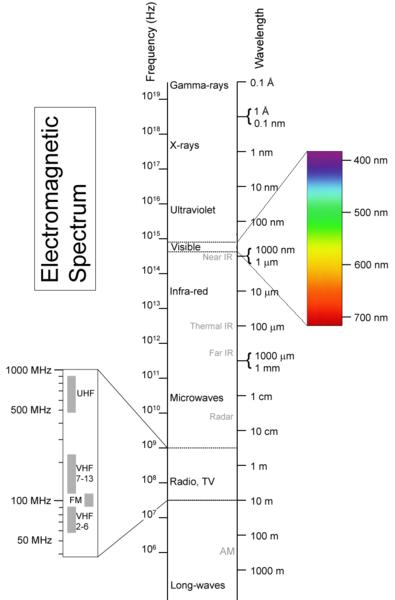This Electrical-Mass then forms an atmosphere around all planets just like the ocean upon the earth.  Light in space = Mass divided by Distance = m/AZ2 = qAZ/AZ2 = q/ZThe exact historically proven equation of the Electrical Ohm (Mass / Time).  And because Albert thought that the velocity of light was a constant rather than "Gravitational Acceleration" being the Constant, he then used the Velocity of Light in his equation rather than using the building blocks of velocity which are (Acceleration x Time).

Albert's equation  E=mc2  declares that the "m" is "Mass as we see it".

E=qA2Z2  or  E=qc2  declares that Albert's "m" is Electrical-Mass (q).

Electrical-Mass Acceleration is the very definition of Electricity.

Electrical-Mass Pressure is the very definition of Gravity.

Electrical-Mass Alignment is the very definition of Magnetism.

The Unification of Energy and Gravity is only possible via the recognition of Electrical-Mass

because it is a building block of all Mass and all Energy.

We can plainly see that when Albert used  (c)  instead of  (AxZ)  he covered up the very factor of Gravitational Acceleration (A) that is present within all Energy.  It's a sad reality but so very true, Willem Gravesande's oversight cost Albert  30 years of computational frustration and untold anguish.  And this all happened because Willem did not realize that his Velocity2 pertained only to the Electrical-Mass portion of the total Mass in his experiment.

AxZ  =  The Speed of Gravity.  The speed of Electricity.

Remember at the beginning of the site, Gravity (A)  x  Orbit Time (Z)  =

The Velocity of Electricity  =  299,792,458 m/s

The Velocity of Accelerated Electrical-Mass.

This equation is also the one and only equation for Electricity.

The true definition of Electricity is

Electrical-Mass  x  Acceleration  x  Distance  along a wire.

Electrical-Mass (q) x Acceleration (A) x Distance (AZ2)  = qA2Z2 = Electricity (Energy).

For those who remain sceptical (time will solve that problem) we can start with the  3  building blocks of Energy.

q-Particle,   Acceleration,   and   Time.

q = Electrical-Mass     A  = Acceleration     Z = Time

(m = Meter, Distance)

It's a well known fact that Electricity is made up of  Light and Heat.  Anyone who has watched a person welding steel will know that the welding machine uses Electricity (Energy) (qA2Z2) to melt steel together.  The welding machine changes Electricity into Light (q/Z) and Heat (A2Z3).Light   x   Heat   =   Electricity.

q/Z   x   A2Z3   =   qA2Z2

If you're at all sceptical;

Rubbing a piece of plastic wrapper between your hands

=

Mass  x  Friction  x  Distance

Mass  x  Force  x  Distance

qAZ  x  qA  x  AZ2

=

q2A3Z3

this then gives you

Distance the hands are rubbed, Heat, an interval of Magnetism (wrapper sticks for a certain length of time), and a Magnetic Field.

=

(Distance) AZ2   x   (Heat) A2Z3   x   (interval of Magnetic Force) qA   x   (length of Time) Z   x   (Magnetic Field) q/AZ3

=

q2A3Z3

and so

q2A3Z3   =   q2A3Z3

Coming to understand that Light x Heat causes Gravity and that aligned Gravity is Magnetism, is exactly parallel to when Galileo back in 1600 a.d. told the people that being at the bottom of the earth would not cause you to fall off into space.  The people of Galileo's time did not understand Gravity therefore to them the earth was flat.  To them when you move to the underside of any object you fall to the floor.  When the people placed their salt shakers upon the bottom of their kitchen tables and then let them go, the shakers fell to the floor.  How could Gravity then possibly make a person stick to the underside of the earth ?  People were imprisoned by their lack of understanding.  To Galileo the earth was round not only because his telescope told him so but because of the very fact that he was able to accept a broader view of Gravity.  So also today, if we do not understand q-Particle Acceleration we will never grasp the complete reality of Gravity and move forward in our understanding of Energy and Electricity.

Be sure to completely understand this reality.

+ 7   x   + 7  =   + 49

- 7    x    - 7   =   + 49

The two mathematical examples above are both correct but only until you add physical entities to them.  As soon as you write;

+ 7 shoes   x   + 7 shoes  =   + 49 shoes

- 7 shoes   x   - 7 shoes  =   + 49 shoes

The  red  equation above is   "half"  right and  half wrong

because there is no such thing as a negative (-) shoe.

Albert Einstein's equation is an exact replica of the red equation above.

It gives a correct numerical answer however its Physics is most certainly incorrect.

When Albert Einstein used "m" as our everyday-Mass in E=mc2 instead of using (Mass / Velocity) he did the exact same thing as using the above red equation instead of the correct blue one.  And because the erroneous red equation gives a correct numerical answer its deception and complete violation of reality has easily gone unnoticed for a long time.

In true and pure Physics there are two Energy equations (below) which give the same numerical answer however they absolutely  cannot  be mixed together.

However Albert mixed the first half of the top equation with the second half of the bottom equation giving us an erroneous hybrid equation that is half right and half wrong and thus the reason that it was impossible for Albert to find unification between his equation and Gravity.  In the exact same way that  -7 x -7  =  + 49.  The  -7  gives a correct numerical result, however  +7  is the True and Pure equation for the very fact that we live in a positive universe.

Because Albert's equation utilizes the inverse (-7) scenario, it completely denies the unification of Energy and Gravity.  The movement of Electrical-Mass (q) is parallel to the (+7) where Albert's movement of Mass (m)  is an exact portrayal of the (-7).  Due to Albert being led astray by the "m" in Willem Gravesande's E=mv2, Albert (surely unknowingly) mixed and combined the two blue segments of the two energy equations below.

The first true Energy equation;

"Mass as we see it" Energy.

Energy = q x (Velocity of Light)2

E = q x (Acceleration x Time)2

E = q(AxZ)2

qAZ = "Mass as we see it"

E = qAZ x AZ     E = mass x Velocity

And so the first true equation is;

Energy  =  mass  x  Velocity

(the exact equation that Isaac Newton gave the world in 1730 a.d.)

The second true Energy equation;

"Electrical-Mass" Energy.

Energy = q particle x (Velocity of Light)2

E = q particle x (c)2

E = qc2

And so the second true equation is;

Energy  =  Electrical-Mass  x  c2

Now when you place the two equations side-by-side;

Energy   =   m                           x   AZ

Energy   =   Electrical-Mass   x   c2

we can see the equation that Albert Einstein gave us;

Energy = mc2

This mixed erroneous hybrid is the  "non-unifiable"  equation

that Albert Einstein gave to the world in  1905 a.d.

The absolute true forms are either;   E = mAZ   or   E = qc2

but  "never"  a combination of the two.

An exactly analogy is found in the photos below.  The outline of the first image parallels Einstein's numerical answer (the outline is correct) however the root, the fundamental core (the shading) of the first image parallels the Physics of Albert's equation and as you can plainly see it is the inverse of reality, the exact inverse of True and Pure physics.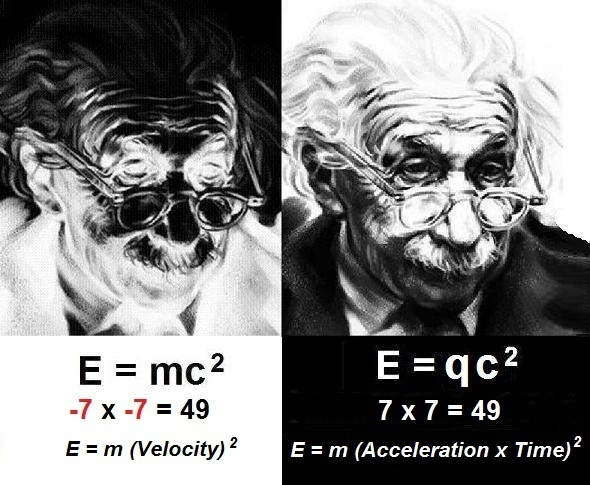The reason that this happened is because Albert saw the speed of light as being a Constant and therefore he did not expect the "factors" that make up Velocity (Acceleration x Time) as being significant enough to be exposed in his equation.  Albert simply did not perceive the fact that the velocity of light is composed of Gravity (Quantum-Mass Acceleration = A) so therefore he just used  "c"  as velocity and saw no reason to reveal its own foundational building blocks of Acceleration and Time.

A perfect analogy is if we had a formula for cookies that was; Dough x Raisins, and we didn't realize that the individual composition of raisins (Grapes x Sunshine) would affect exactly what the formula portrays, we wouldn't then write the formula as;  Dough x (Grapes x Sunshine).  And this is exactly why Albert was unable to connect Gravity to his equation because he covered up Gravity (Acceleration) by only using the broad unit of Velocity (c).

A monumental law in True and Pure Physics that Albert Einstein did not realize is that equations that are not reduced to their smallest possible factors will always contain and enable an

equal yet inverse half-correct solution.

Here's an exact example.  The formula for the kite below is;

Flight  =  kite  x  Velocity of wind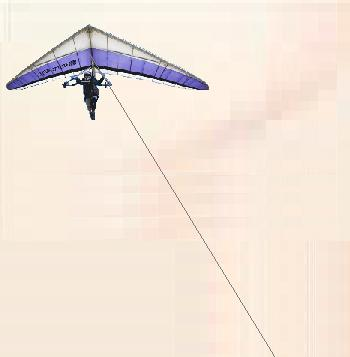The equation above and the image that portrays it, possesses two whole and separate solutions because the equation has not been reduced to its smallest possible factors.  This equation can either portray;

1.   A stationary tethered kite with air moving past it.   or

2.   A moving kite being propelled through stationary air.

One of the above is the true "realm" of the Physics and the other is the inverse half correct solution that occurs as a result of the equation not being reduced to (Acceleration x Time).  Yet both produce the same solution of Flight.

We cannot tell whether the kite is tethered or whether it is being propelled.  We cannot tell if the air is moving past the kite  "or"  if the kite is moving past stationary air.

Due to the fact that the equation has not been reduced to its smallest factors, the specific  "realm"  of the physics is not determined and so the equation then enables two possibilities.  However the Flight (the left side of the equation) the numerical answer to both possibilities will be the same for both realms 1 and 2.  Flight occurs whether the kite is moving or whether the air is moving past the kite.  This is why Einstein's equation gave a correct "numerical" answer for energy and yet an incorrect "realm" answer for the very Physics of Energy.

And it's because of this fact that un-reduced formulas provide two equal yet opposite possible realms that Albert Einstein's error has been hidden for so long.  The error has been hidden because E=mc2 gave a correct answer for the numerical value of energy and yet it gave a completely in-correct value for the realm of the actual Physics.

However now when we reduce the equation and write it in its true format;

Flight  =  kite  x  (Acceleration x Time)

the Acceleration is now directly connected to the kite.  The above equation absolutely declares that for a period of Time the kite Accelerated.  And when we write the equation in this manner the image, the specific realm of the physics, is then transformed into this;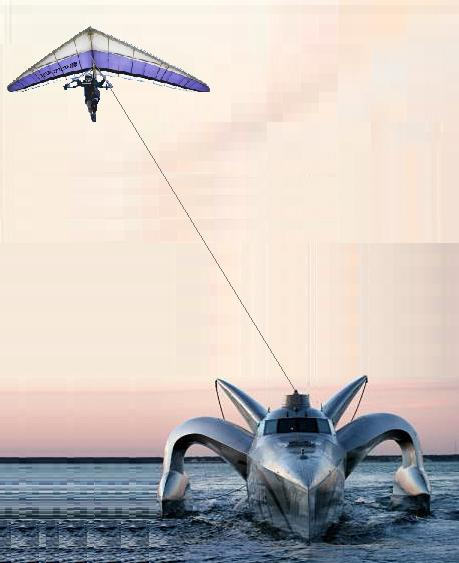We now know for fact that the kite is moving past the stationary air as opposed to the line being tethered and the air moving past a stationary kite.  If the kite was tethered the equation would be;

Flight  =  Kite  x  (Air x Acceleration x Time)

And hence with the Pure and True equation of the Acceleration of the kite we can then unify the Flight of the Kite with the Boat in a way that we were unable to do in the first image.  Albert Einstein could not unify his energy equation with Gravity because his use of Velocity kept the very  "realm"  of the physics of Gravity a mystery.

When the two kite equations are then placed beside the two Energy equations we can clearly see how their compared realms of Physics are exactly parallel.

The incorrect equations that also provide inverse possibilities.

Flight  =  kite  x  Velocity of wind

(possesses the inverse possible realm of air moving past a tethered kite)

Energy  =  mass  x  Velocity2     (E=mc2)

(possesses the inverse possible realm of Mass being Energy)

The correct equations that portray the true physical realms.

Flight  =  kite  x  (Acceleration x Time)

(declares for certainty that the kite is moving past stationary air)

Energy  =  mass  x  (Acceleration x Time)2

(declares for certainty that Light "within" Mass is Energy)

The Truth Is;

Energy = Electrical-Mass x (Speed of Gravity)2

Speed of Gravity = Speed of Electricity

Speed of Gravity = The Acceleration of Gravity x Orbit Time

Electrical-Mass Acceleration = Energy

(the exact inverse of Einstein's mass = Energy)

Mass does not = Energy.   Accelerated Electrical-Mass "within" all Mass = Energy.

Gravity = The Acceleration of Electrical-Mass  =  Electrical Pressure.

The Acceleration of Electrical-Mass  x  Distance  "is"  Electricity.

The Speed of Electricity  =  The Speed of Gravity

Light and Heat are the core of Electricity.   Light and Heat are the core of Gravity.

Light and Heat are the core of Magnetism

E=mc2  =  Energy   but   Numerically Only !

Einstein's mass x c2 could never lead us to truly defining Gravity.

Electrical-Mass Pressure   "is"   Gravity.

Electrical-Mass is the root of   "all"   forms of Energy.

(Electricity, Magnetism, Gravity, Combustion, Radiation, . . . etc. )

E = qA2Z2

q, A, and Z, are the keys to our physical universe.

Gravity is  not  a pulling force.

Gravity is absolutely a  pushing  force of Electrical Pressure.

A Light Wave is just a  2 dimensional view of a 3 dimensional helix.

The Particle's Frequency x Wavelength.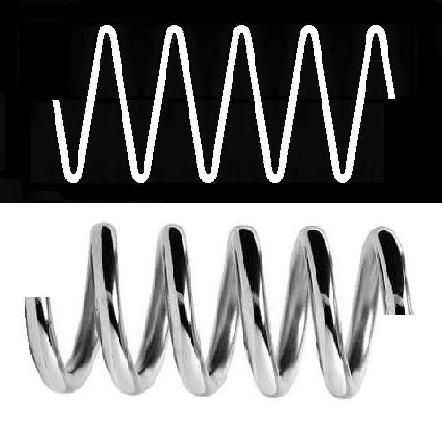A  Wave  is the 3 dimensional path of a Particle.

Waves and Particles are "one" hence the solution to the long perplexing double slit experiment.

Why is Truth and Discovery so often faced with great  "opposition"   from the human race   ?

Just this morning (November 22/09) we heard on the news that an Italian Doctor (Paolo Zamboni) made an astounding breakthrough discovery in the science of Multiple Sclerosis.  Dr. Zamboni has just discovered that  "only"  in the neck veins of MS patients, there occurs a twist in the vein which then causes blood (iron) to backup into the brain which then causes what we know as the disease of Multiple Sclerosis (MS).

And already members of the human race are denying Dr. Zamboni's giant discovery and great work.  Let us repeat, this rare twisting of the vein within the neck is only found in patients who are diagnosed with MS.

Are we as people of the human race also diseased by a lack of common  LOGIC ?

Sadly, Dr. Zamboni's discovery is yet another incredible example of inopportune opportunity for human opposition to Truth and Discovery.

Galileo (1600)

It was not the authorities who refused to look through Galileo's telescope. It was his fellow scientists !  They declared that using a telescope was a waste of time, for even if they did see evidence for his claims, it would only be because Galileo had unfairly bewitched them as he dare propose the evil Copernican viewpoint that the sun was the center of our galaxy.

William Harvey (1630)

The first western scientist to describe the circulatory system with the heart as its central pump.  Unfortunately he described it in the early 17th century and was nearly drummed out of the scientific community for doing so.  Yet he fared far better than Servetus who described the pulmonary circulatory system in the 1500's and was rewarded by being burned at the stake.

Andre Ampere (1800)

For 10 years the famous Mr. Andre Ampere's work was ridiculed and ignored including his grand discovery that force between current elements does not obey a simple inverse square law.  Today his name is the root of Electricity.

George Ohm (Ohm's Law) (1830)

Ohm's initial publication was met with ridicule and dismissal.  His work was called "fantasy".  Ten years passed before scientists finally recognized its great importance but definitely not before Ohm was forced to resign his job as a high school teacher.  After 10 years of “resistance”, the scientific community finally figured out that Ohm's law was absolutely correct.

Julius Mayer (The Law of the Conservation of Energy) (1850)

Mayer's original paper was contemptuously rejected by the leading physics journals of the time.  Guess what, Energy can neither be created nor destroyed.  Man can neither create nor destroy; Mass, nor Gravity, nor Distance.

Nikola Tesla  (King of all Physicists) (1900)

Tesla was greatly ridiculed for his claim that the whole earth would resonate electrically at 7Hz, 14Hz, 21Hz, etc. all the way up into the tens of kilohertz.  He claimed to discover this phenomenon during his radio observations of lightning strikes.  The physicists of the time would have nothing to do with it.  Decades later in the 1950's after Tesla was safely dead, during investigations of the VLF radio signals produced by lightning it was discovered that indeed the whole earth resonates electrically at  7Hz, 14Hz, etc.

Jacobus Van't Hoff (Theory of 3D molecules) (1900)

As a relative newcomer and quite unknown, Jacobus was attacked and ridiculed for proposing that a 3D tetrahedral structure would explain many problems in chemistry.  His foes rapidly went silent as his ridiculous cardboard models won the first Nobel Prize in chemistry.

Barbara McClintlock (Transposons) (1983)

Barbara finally won the Nobel Prize in 1984 after enduring 32 years harsh ridicule and being ignored for her work on the mobility of genetic sequences of DNA that move to different positions within the genome.

"The only time that Physics will lie to us is when we don't believe that units math is Math.
Then via our disbelief we indeed dishonor math however math will never give up on us.
Mathematics is the one who refuses to give up on us as it tenaciously summons us.
Calmly and unceasingly beckoning us in hopes that it just may somehow waken us from our . . .

intellectual slumber."

For further reading click here.

 Name (Symbol) Base Unit Unit Symbol Angular Frequency radians/second ω Admittance (Y) siemens S Capacitance (C) farad F Capacitive Reactance (XC) ohm Ω Center Frequency (Fc) hertz Hz Charge coulomb C Conductance (G) siemens [mho] S  [1/Ω] Conductivity (σ) siemens/meter S/m Current (I) ampere A Dipole Moment coulombÂ·meter p Electric Charge coulomb Q Electric Flux coulomb Ψ Electric Flux Density coulomb/meter2 D Electric Susceptibility 1 χe Energy (E) joule J Frequency hertz Hz Impedance (Z) ohm Ω Inductance (L) henry H Inductive Reactance (XL) ohm Ω Joule watt·second J Linear Current Density amperes/meter2 A Magnetic Field tesla T Magnetic Flux weber Φ Magnetic Flux Linkage weber Λ Magnetic Flux Density tesla B Magnetic Susceptibility 1 χμ Magnetic Vector Potential weber/meter A Magnetization ampere/meter M Magnetomotive Force weber Wb Mutual Inductance henry Li,j    Mi,j Permeability henrys/meter μ Permittivity farads/meter ε Phase Angle radian Φ Phase Coefficient radian β Potential (V) volt V Power (P) watt W Reluctance (R) 1/henrys 1/H Reluctivity meters/henry ν Resistance (R) ohm Ω Resistivity (ρ) ohm·meter Ω*m Resonant Frequency (Fr) hertz Hz Surface Density of Charge coulombs/meter2 σ Susceptance (B) siemens S Volume Density of Charge coulombs/meter3 ρ Volume Resistivity ohms/meter3 ρ Wavelength meter λ

The Unification of Gravity with Einstein’s  E=mc2

was discovered by  AZ

See the complete and amazing story at

©  Copyright All Rights Reserved  EinsteinGravity.com  2008